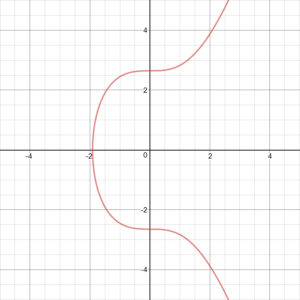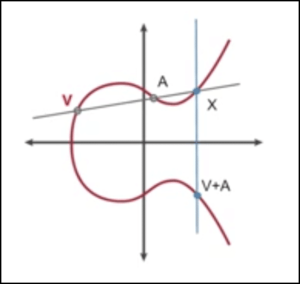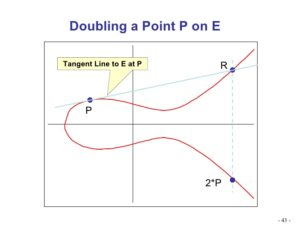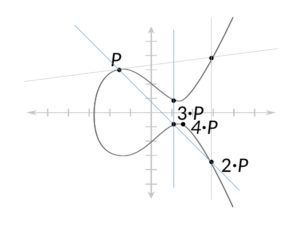# What Is Elliptic Curve Cryptography? Technology Behind Digital Signatures in Cryptocurrencies

Cryptography underpins the digital signature schemes of cryptocurrencies and is the basis for their secure transaction verification between two parties across a decentralized network. There are numerous cryptographic methods used by different cryptocurrencies today, focusing on providing efficient and secure transaction models.

Elliptic Curve Cryptography (ECC) is one of the most widely used methods for digital signature schemes in cryptocurrencies, and a specific scheme, the Elliptic Curve Digital Signature Algorithm (ECDSA) is applied in both Bitcoin and Ethereum for signing transactions.## Background of ECC and ECDSA

Elliptic Curve Cryptography was suggested by mathematicians Neal Koblitz and Victor S Miller, independently, in 1985. While a breakthrough in cryptography, ECC was not widely used until the early 2000’s, during the emergence of the Internet, where governments and Internet providers began using it as an encryption method.

Compared to RSA encryption, ECC offers a significant advantage. The key size used for ECC is much smaller than what is needed for RSA encryption, while still providing the same level of security. Although RSA encryption is more widely used across the Internet today, ECC is essentially a more efficient form of RSA, which is one of the primary reasons it is used in cryptocurrencies.Read: What is RSA Cryptography? Complete Guide to this Encryption Algorithm

The U.S. National Institute of Standards and Technology (NIST) endorses ECC as its “Suite B” recommended algorithms, and the NSA officially supports classifying top secret information with 384-bit keys. As an example of the efficiency of ECC as compared to RSA, the same 384-bit key used in encrypting classified information would require a 7680-bit key using RSA encryption. The efficiency afforded by ECC is therefore exceedingly useful to blockchain networks since it reduces the size of transactions.

## How Does It Work

Elliptic Curve Cryptography is a method of public-key encryption based on the algebraic function and structure of a curve over a finite graph. It uses a trapdoor function predicated on the infeasibility of determining the discrete logarithm of a random elliptic curve element that has a publicly known base point.

Trapdoor functions are used in public-key cryptography to make it so, going from A —> B is trivial, but going from B —> A is infeasible, by leveraging a specific mathematical problem. For instance, RSA encryption is based on the concept of Prime Factorization, and ECC relies on the concept of Point Multiplication, where the multiplicand represents the private key and is infeasible to compute from the given starting points.

The elliptic curve needs to consist of points that satisfy the equation:

y^2 = ax^3+ b

(x, y) on the curve represent a point, while both a and b are constants. Theoretically, there are infinite curves that could be created, but specifically applied to cryptocurrencies (in the case of Bitcoin and Ethereum), a particular elliptic curve called secp256k1 is used. It is represented in the image below.As you can see, elliptic curves are symmetric about the x-axis. Due to this, if you draw a straight line starting from a random point on the curve, the line intersects the curve at no more than 3 points. You draw a line through the first two points and determine where the line intersects with the third point. Next, you reflect that third point across the x-axis (it’s symmetrical) and that point is the result of adding the first two points together. This is demonstrated in the image below.In the graph above, V and A represent the starting points, X represents the third point, and the final point (let’s call it Z) represents adding V and A together. When used in a digital signature scheme, the base point of the line is typically predefined.

For ECC to create a trapdoor function, elliptic curve cryptography uses point multiplication, where the known base point is repeatedly added to itself. In such a case, let’s use a base point P, where the goal is to find 2P, as outlined below.Above, a tangent runs from point P through point R, which is the intersection point. The reflection of that point is 2P. Suppose we want to continue this and to find 3P, 4P, and so on. Next, we join P and 2P and subsequently reflect this point over the intersection, and continue to do this for 4P. Illustrated below:This is the multiplicative property of the graph because we are finding points that are a multiplication of an integer with the point itself. The result is what gives the function its trapdoor, known as the discrete logarithm problem.

If we represent a variable x as a 384-bit integer and multiply it with the base point P, the result is a point on the curve, called Z. Applied to cryptocurrencies, Z is public, but the original variable x is secret (private key). To determine x from Z and P, you would need to determine how many times P was added to itself to get the point Z on the curve. This problem is a form of modular arithmetic that is mathematically infeasible and is why ECC is so secure.

## Use In Cryptocurrencies

When analyzing the need for digital signatures schemes in cryptocurrencies, there are 4 primary requirements of any given scheme that must be met for the signature scheme to be provably authentic and verifiable. These include:

1. It should be provably verifiable that a signer of a transaction is the signer.
2. The signature should not be forgeable.
3. The signature needs to be non-repudiable, meaning signatures are final and cannot be associated with another identity.
4. It should be computationally infeasible to derive the private key from a corresponding public key.

Elliptic Curve Cryptography satisfies all 4 conditions and is also particularly effective in doing so. Using ECC, the (x, y) coordinates of a point on the graph would be your public key, and the 384-bit random integer x would be your private key.

It is also possible to prove to somebody that you know the value of x, without actually revealing what x is. This property further helps to satisfy the necessary conditions for sustainable use in a digital signature transaction scheme.

## Quantum Concerns

The use of ECC in digital signature schemes for cryptocurrencies is exceptionally secure. However, concerns have been raised recently regarding the future potential of quantum computers and their substantial power having the ability to break ECC. Although its possibility is considered to be years away, Shor’s algorithm would theoretically be able to compute discrete logarithms on a hypothetical quantum computer with sufficient power.

Various cryptocurrencies have taken a forward-thinking approach to the potential threat raised by quantum computers by implementing quantum-resistant algorithms as the foundation of their digital signature schemes. Even the NSA in 2015 announced its planned future transition away from ECC, and to a different suite of ciphers for its encryption needs due to the looming inevitability of quantum computing power.Read: Quantum Computing: What Threat Does It Pose to Bitcoin?

These concerns are primarily speculation at this point, as the quantum computing power necessary for Shor’s algorithm to compute discrete logarithms is substantially higher than even the most powerful early-stage quantum computers in existence today.

## Conclusion

Looking ahead, successive generations of cryptocurrencies may eventually transition to more advanced encryption methods for securing their transactions, and potentially Bitcoin and Ethereum may need to make the same transition.

For now, ECC and other digital signature schemes utilizing trapdoor functions remain some of the most secure encryption methods in the world and should continue to remain so for some time.

The post What Is Elliptic Curve Cryptography? Technology Behind Digital Signatures in Cryptocurrencies appeared first on Blockonomi.